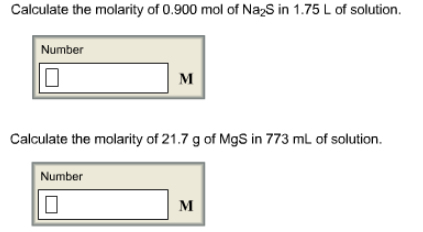# Problem: Calculate the molarity of 0.900 mol of Na2S in 1.75 L of solution.Calculate the molarity of 21.7 g of MgS in 773 mL of solution.

###### FREE Expert Solution
91% (276 ratings)###### Problem Details

Calculate the molarity of 0.900 mol of Na2S in 1.75 L of solution.

Calculate the molarity of 21.7 g of MgS in 773 mL of solution.